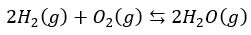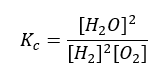# Law of mass action

Equilibrium: Law of mass action

The Law of Mass Action states that the equilibrium expression depends on the equilibrium concentrations of reactants and products. When a reactive system comes to equilibrium at a given temperature, the value of equilibrium constant is always the same even when all concentrations are different. This constant value of equilibrium is called the equilibrium constant, K.This means that the value of the equilibrium constant expression K is constant for a reaction at a given temperature, whenever equilibrium concentrations are substituted. The Law of Mass Action holds whether the reaction is elementary or not.

Each “reactive system” can be defined by a balanced chemical equation. Consider a reaction where reactants A and B react with each other to produce products C and D with a,b,c and d being the respective number of moles present in the system. Here, it does not matter what initial amounts you start within your system, some net reaction will occur until equilibrium is established.

Consider the following generalized reactionFigure 1: Generalized reaction equation

For dilute solutions, the law of mass action describes the equilibrium mass distribution

K = (C)c(D)d/(A)a(B)b

where K is the equilibrium constant and (A),(B),(C), and (D) are the molal (or molar) concentrations. Here, the value is constant for a given reaction systemat a given T which means that K varies with T. The value of K can be between 0 and ∞ but it cannot be zero in a dynamic equilibrium state. The initial and final distribution or relative amounts of the reactant and products does not matter as the relationship remains true.

In non-dilute solutions, ions interact electrostatically with each other. These interactions are modelled by introducing activity coefficients (γ) to adjust molal or molar concentrations to illustrate effective concentrations

[A] = γa(A)

Activities are usually smaller for multivalent ions than for those with a single charge

The law of mass action can now be written:

K = γc(C)c γd(D)da(A)a γb(B)b=[C]c[D]d/[A]a[B]b

The Law of Mass Action says nothing about the rate at which equilibrium will be established. It can be extremely slow or extremely fast and is not related to the value of K. The tendency of the reaction is not equivalent to its rate.

Consider the following reaction:The steps to construct the equilibrium law equation are:

Step 1: Set up the “equilibrium law” reaction equation.

Step 2: Product concentrations go in numerator

Step 3: Concentration in the expression is raised to the coefficient of the product

Step 4: Reactant concentrations go in the denominator

Step 5: Concentrations in the expression are raised to the coefficients of reactants

State (g, l, s, aq) may or may not be added at this point butthe value of Kc will depend on temperature, thus this is listed along with the Kc value. Also, the equilibrium law includes concentrations of products and reactants in mol/L (M).

Likewise, the mass action expression is written as:Where Kc is the equilibrium constant.

.